# 点积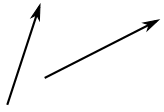## 运算

a · b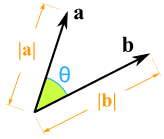a · b = |a| × |b| × cos(θ)

|a| 是 矢量 a 的量值
|b| 是 矢量 b 的量值
θ 是 ab 之间的 角度

a 的长度 乘以 b 的 长度，再乘以 ab 之间的角的余弦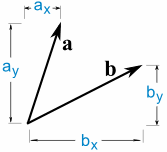a · b = ax × bx + ay × by

### 例子：求 a 和 b 的 点积：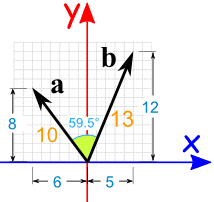a · b = |a| × |b| × cos(θ)

a · b = 10 × 13 × cos(59.5°)
a · b = 10 × 13 × 0.5075……
a · b = 65.98…… = 66 （舍入后）

a · b = ax × bx + ay × by

a · b = -6 × 5 + 8 × 12
a · b = -30 + 96
a · b = 66

## 为什么用 cos(θ) ？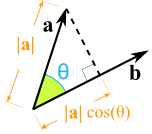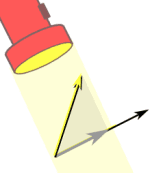我们用与 b 方向相同的 a 的部分 好像用光照着来找影子一样

 如果我们把 b "投影" 到 a，然后相乘，得出来的答案将会是一样的： 因为点积乘法的次序并不重要： |a| × |b| × cos(θ) = |a| × cos(θ) × |b|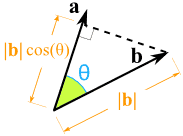## 直角

### 例子：求以下矢量的点积：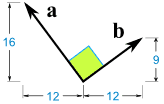a · b = |a| × |b| × cos(θ)

a · b = |a| × |b| × cos(90°)
a · b = |a| × |b| × 0
a · b = 0

a · b = ax × bx + ay × by

a · b = -12 × 12 + 16 × 9
a · b = -144 + 144
a · b = 0

## 三维或更高

### 例子：璐璐测量了两根旗杆的尖端的位置，她想知道它们 之间的角度：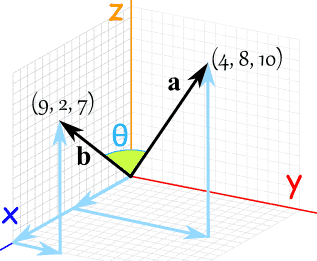a · b = ax × bx + ay × by + az × bz

a · b = 9 × 4 + 2 × 8 + 7 × 10
a · b = 36 + 16 + 70
a · b = 122

a · b = |a| × |b| × cos(θ)

|a| 是什么？它是矢量 a 的量值（长度）。我们可以用 勾股定理来求：

• |a| = √(42 + 82 + 102)
• |a| = √(16 + 64 + 100)
• |a| = √180

|b| 也一样：

• |b| = √(92 + 22 + 72)
• |b| = √(81 + 4 + 49)
• |b| = √134

a · b = |a| × |b| × cos(θ)

122 = √180 × √134 × cos(θ)
cos(θ) = 122 / (√180 × √134)
cos(θ) = 0.7855……
θ = cos-1(0.7855……) = 38.2……°